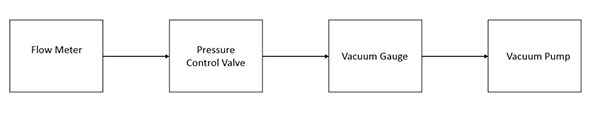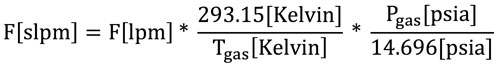# Vacuum Pump Testing Method

## Standard method for testing Air Squared scroll vacuum pumps with air.

Air Squared uses NIST-calibrated equipment for all testing practices. For open-loop (air) testing, the test bench is assembled as shown in Figure 1. Arrows represent the flow of gas through the system, ending at the inlet of the pump. For closed-loop testing with gases other than air, contact Air Squared to discuss test bench requirements.Figure 1: Vacuum Pump Test Assembly

#### Test Stand

Required materials for a scroll compressor test stand are:

• Flow Meter
• Amp Meter
• Multimeter or Volt Meter
• Pressure Gauge
• Pressure Control Valve
• Tachometer or Rate Meter
• Laser Thermometer
• Cooling Fan
• Performance Datasheet

Reference a detailed scroll vacuum pump test bench schematic using the following link:

Test Bench Schematic

#### Measurements

Standard recorded measurements are: date, time, motor speed, inlet pressure (vacuum), inlet temperature, ambient pressure, ambient temperature, flow rate (upstream of valve – see schematic), voltage, and current.

##### Flow

Air Squared publishes inlet volume flow at NIST standard ambient conditions. Although there is variance among vacuum pump manufacturers, most publish inlet volume flow at standard ambient conditions, as opposed to the reduced pressure (vacuum) condition. The ambient pressure condition is constant, whereas the vacuum pressure condition changes the density of the fluid as pressure changes.

Air Squared publishes vacuum pump flow in liters per minute (lpm) or cubic feet per minute (cfm). The industry also references standard liters per minute (slpm) or standard cubic feet per minute (scfm). This means the flow rate is corrected for NIST standard temperature and pressure (STP) – 101.325 kPa and 293.15 Kelvin. The equation for correction is shown in Figure 2.Figure 2: Standard Liter per Minute (slpm) Correction Formula

Air Squared considers a volume flow rate reported at standard conditions (slpm or scfm) a mass flow rate. Technically speaking, a standard flow rate (slpm or scfm) is a volume flow rate (lpm or cfm), albeit one corrected to STP. However, at STP, the fluid density is known, so the mass flow rate is easily calculated. Thus, standard volume flow rate and mass flow rate are used interchangeably, and the meaning is still understood. Regarding units, volume per time is always a volume flow rate and mass per time is always a mass flow rate, but standard volume per time can be referenced as either volume flow at STP or mass flow (as density is known at STP).Figure 3

Air Squared measures flow using a mass flow meter. A mass flow meter is superior to a rotameter, as a rotameter is typically calibrated to a specific condition (primarily STP), and any significant deviation from that condition greatly reduces accuracy. The ambient pressure in Broomfield, CO is much lower than standard ambient pressure, so a rotameter calibrated to STP is ineffective.

There is generally a pressure drop across the flow meter, so it is placed upstream from the pressure control valve. The flow rate at any location within the system can be found using the equation in Figure 3.

##### Power Consumption

Motor power consumption is measured using a multimeter (voltage) and an amp meter (current) as close to the motor as possible. With brushless DC motors (BLDC), power is best measured at the appropriate motor leads between the controller and the motor.

##### Speed

Speed is measured either manually using a tachometer directly on the motor shaft or an available bearing, or electronically using an RPM gauge that counts the motor pulses.

##### Temperature

Gas temperatures are measured using fluid thermocouples. Surface temperatures are measured using a laser thermometer on the front face of the vacuum pump, as close to the discharge port as possible (location of maximum temperature).

#### Operation

Compression (including a vacuum pump – vacuum condition compressing to the ambient pressure) is an exothermic process, with excess heat managed using a cooling fan (or liquid cooling) to force convection. Air Squared always recommends the use of additional cooling when available.

Data points are measured and recorded only after the compressor has achieved steady-state temperature. Steady-state temperature is dependent on the local environment, so steady-state is assumed to use the standard cooling fan and ambient conditions in Broomfield, CO at the time of testing. Steady-state temperature is confirmed by measuring the surface temperature of the vacuum pump, at the same location, in periodic intervals until constant.

#### Altitude

Air Squared is located in Broomfield, CO – 1,640 meters (5,380 feet) above sea level. The atmospheric pressure at this altitude is approximately 0.835 bara (12.1 psia). All Air Squared air vacuum pumps are tested at this altitude, so the atmospheric pressure differential between Broomfield, CO and sea level should be considered.

For vacuum pumps at a given speed, the lower ambient air density in Broomfield, CO can result in lower power consumption and a lower flow rate, than testing at sea level, to reach the same absolute pressure, but higher power consumption and a higher flow rate, than testing at sea level, to reach the same gauge pressure.

September 28, 2015